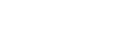# Loan Calculator through VBA

In this article, we are going to learn how to create calculator to calculate the Interest amount through VBA in Microsoft Excel.

User Form: - User form is used to enter the specific details in the data, which is basically used by the users who are not friendly with the Excel. Through user form, we can restrict the entries and assign the cells in which that particular entry is required.

We want to create a user form to enter the few details and then Excel will calculate the Interest amount.Follow below given steps to create the User form for Interest amount calculation

• Insert a User Form, then insert 3 label boxes, 3 text boxes and one command button• Double click on Command button to assign the Macro
• Write the below-mentioned code:-
```Private Sub CommandButton1_Click()
Dim Amount, rate, duration, interest As Single
Dim erow As Integer
erow = Worksheets("Sheet1").Cells(Rows.count, 1).End(xlUp).Offset(1, 0).Row
Amount = Val(TextBox1.text)
Cells(erow, 1).Value = Amount
rate = Val(TextBox2.text) / 100
Cells(erow, 2).Value = rate
Cells(erow, 2).Value = Cells(erow, 2) * 100 & "%"
duration = Val(TextBox3.text)
Cells(erow, 3).Value = duration
interest = ((Amount * (1 + rate) ^ duration) - Amount)
Cells(erow, 4).Value = interest
End Sub
```Code Explanation: - First, we will define the variables, and then we will define rows and cells where we want to capture the details in the text box.

Now, we will insert a button to open the user form, follow below given steps:-

• Go To Developer tab > Controls group > Insert > Button (form control)• Insert the button on sheet
• After inserting the button on sheet, “Assign Macro” dialog box will open• Click on New
• Visual Basic Editor will open
• Write the code mentioned below
```Sub Button5_Click()

Interest_Cal.Show

End Sub
```• When you will click on button, calculator form will appear• In which we have to enter amount, then %age, and then Duration
• When you will click on Command button (Calculate), entries will get entered to the defined cells and will calculate the interest amount in D columnIn this way, we can create the loan calculator in Microsoft Excel by using VBA coding in Microsoft Excel.